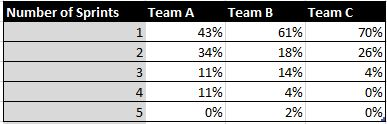# Number of Sprints for issues to get completed

Hi,
I want to create a report that shows me the number of sprints that issues (stories and tasks) took to get completed.
So the report should list x% of the issues have been completed within 1 sprint, y% have been completed within 2 sprints and so on.I’m struggeling on how to create a calculated member that shows the number of sprints used to complete an issue.

Also interested in this.

I am also interested in this type of report

Hi everyone,

Not sure if this is what you expected but maybe it will give you some good ideas.

[Issues completed up to now %]

``````CASE
WHEN
[Sprint].CurrentHierarchyMember.Level.Name = "Sprint"
THEN
Sum(
{[Sprint].CurrentHierarchyMember.Parent.FirstChild:
[Sprint].CurrentHierarchyMember},
[Measures].[Issues resolved]
)
ELSE
[Measures].[Issues resolved]
END
``````

[Issues completed up to now %]

``````CASE
WHEN
[Sprint].CurrentHierarchyMember.Level.Name = "Sprint"
THEN
Sum(
{[Sprint].CurrentHierarchyMember.Parent.FirstChild:
[Sprint].CurrentHierarchyMember},
[Measures].[Issues resolved]
) /
([Sprint].CurrentHierarchyMember.Parent,
[Measures].[Issues resolved])
ELSE
1
END
``````

Gvido Neilands, flex.bi

Hi,
This is not really what I’m looking for, but gives interesting data that I can use for my project too!What I’m looking for is:
We have x stories completed.
How many of them have been completed within 1 sprint, how many took 2 sprints, how many took 3 sprints and so on.
So I want to see how good the team is in achieving the goal of finishing stories within 1 sprint.
This query should not be limited to a single sprint but e.g. for the last 6 months

Does this make it clearer?

Thanks, Andreas

Hi,

Very recently I sent an email to the eazyBI team and, for something related to this I got help with this formula:

case when
[Issue].Currentmember.Level.Name = “Issue”
Then
Generate(
Filter(
[Sprint].[Sprint].Members,
[Sprint].CurrentMember.Name <> “(no sprint)” and
[Measures].[Transitions to] > 0
),
[Sprint].CurrentMember.Name, ", "
)
End

This shows also sprints in which an issue has been, and the following counts them:

case when
[Issue].Currentmember.Level.Name = “Issue”
Then
Count(
Filter(
[Sprint].[Sprint].Members,
[Sprint].CurrentMember.Name <> “(no sprint)” and
[Measures].[Transitions to] > 0
))
End

This is close I could get to what I needed, and it’s very useful.

It would be more convenient to have a separate dimension Number of Sprints to analyze how many Sprints it took to work on an issue. You may build such dimension using JavaScript calculated custom fields.

``````# Number of Sprints
[jira.customfield_sprintCount]
name = "Number of Sprints"
data_type = "string"
dimension = true
javascript_code = '''
if(issue.fields.customfield_NNNNN){
issue.fields.customfield_sprintCount = issue.fields.customfield_NNNNN.length
};
'''
``````

, where NNNNN is field “Sprint” ID in Jira.

2)Then in import options, select custom field Number of Sprints for data import as property and as dimension.

3)Report to analyze issue rate based on sprint count assigned to an issue may look like this (see picture below).

You may define calculated measure for rate based on Issues created, Issues Resolved or other measure, like this:

``````[Measures].[Issues created]
/
([Measures].[Issues created], [Number of Sprints].DefaultMember)
``````

Best,
Zane / support@eazyBI.com

2 Likes

Hi

I’ve found this thread useful and I’ve add the ‘Number of Sprints’ custom field definition into my workspaceI would like to be able to produce a report that for each project, could list the average number of sprints that it takes for all issues to be closed. Is this possible?

example…

Project A 2.5
Project B 1
Project C 3.75
Project D 1

Whilst I’m at it…I’d also be interested how I could show the number of issues added to or removed from a sprint once a sprint has started.

The icing on the cake would be if I could also show the sum of the time spent on issues during the sprint start and end date, but where the issue was not in the sprint. I’d pay good money for this

example

Sprint x +5 issues 22 hours
Sprint y -2 issues 6 hours
Sprint z +6 issues 45 hours

Hi ,

I tried to use the code of “Number of Sprints” and it shows exactly what I see in Jira on the sprints list but the problem is that there is bug in Jira side - if story moved from sprint to sprint it won`t appear in this list (while in its history I will see it) .

I wrote calculable which give me the number of sprints which issue visit (active sprints) :

case when
[Issue].Currentmember.Level.Name = “Issue”
Then
Count(
Filter(
[Sprint].[Sprint].Members,
[Measures].[Issue In Sprint] = 1
))
End

Above use “Issue In sprint” calculable
– return if issue was in sprint or not
CASE WHEN ([Measures].[Sprint Story Points committed] > 0 OR
[Measures].[Sprint Story Points added] > 0 OR
[Measures].[Sprint Story Points removed] <> 0 OR
[Measures].[Sprint Story Points change] <> 0) THEN
1
END

Will appreciate if you can advise how can I change the code of “Number of sprints” to support it .